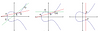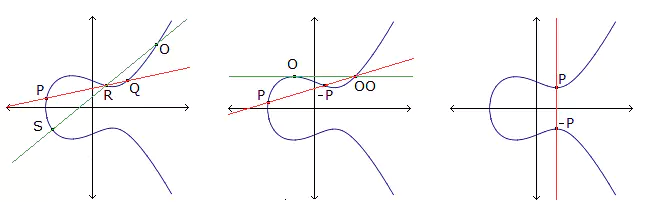# Some Combinatorial Aspects of Constructing Bipartite-Graph Codes

### Journal article

A. Davydov, M. Giulietti, S. Marcugini, F. Pambianco
Graphs Comb., 2009

Semantic Scholar ArXiv DBLP DOI
Cite

### Cite

APA
Davydov, A., Giulietti, M., Marcugini, S., & Pambianco, F. (2009). Some Combinatorial Aspects of Constructing Bipartite-Graph Codes. Graphs Comb.

Chicago/Turabian
Davydov, A., M. Giulietti, S. Marcugini, and F. Pambianco. “Some Combinatorial Aspects of Constructing Bipartite-Graph Codes.” Graphs Comb. (2009).

MLA
Davydov, A., et al. “Some Combinatorial Aspects of Constructing Bipartite-Graph Codes.” Graphs Comb., 2009.

## Abstract

We propose geometrical methods for constructing square 01-matrices with the same number n of units in every row and column, and such that any two rows of the matrix contain at most one unit in common. These matrices are equivalent to n-regular bipartite graphs without 4-cycles, and therefore can be used for the construction of efficient bipartite-graph codes such that both the classes of its vertices are associated with local constraints. We significantly extend the region of parameters m, n for which there exist an n-regular bipartite graph with 2m vertices and without 4-cycles. In that way we essentially increase the region of lengths and rates of the corresponding bipartite-graph codes. Many new matrices are either circulant or consist of circulant submatrices: this provides code parity-check matrices consisting of circulant submatrices, and hence quasi-cyclic bipartite-graph codes with simple implementation.

Share